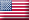# Set up a payroll formula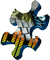If you are completing the Sage 50 Practice Set, you are asked to set up a basic payroll formula in Sage 50.

Once you have completed the Payroll Setup wizard and you are running payroll yourself instead of using the full service payroll solution provided by Sage, the next step is to set up your own payroll formulas that Sage 50 will use to calculate various payoll taxes and liabilities for your business. Note that if you subscribe to Sage's full payroll solution, you will be automatically provided with up-to-date formulas that you can use to process your payroll transactions. However, it is useful to understand the process of setting up a payroll formula in Sage 50 so that you know how to do this should the need arise. This topic shows you where and how to set up a payroll formula in Sage 50. It also shows you how to create a basic equation that is used to calculate the result of a payroll formula in Sage 50.

To set up a payroll formula, select the File menu and choose Payroll Formulas and then User-Maintained from the options available.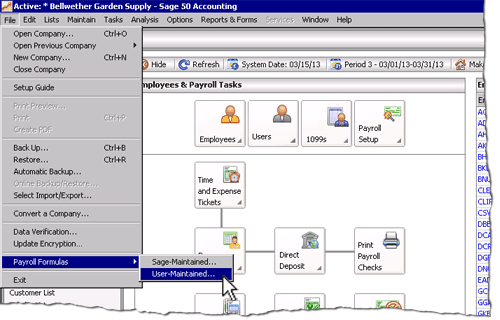This opens the User-Maintained Payroll Formulas window where you can enter information about a payroll formula into Sage 50. The following screenshot and reference table show step-by-step instructions on how to set up a simple payroll formula using the User-Maintained Payroll Formulas window. Note that each step is assigned a reference number to help you identify the appropriate field or icon in the screenshot of the User-Maintained Payroll Formulas window.

#### The User-Maintained Payroll Formulas window with reference numbers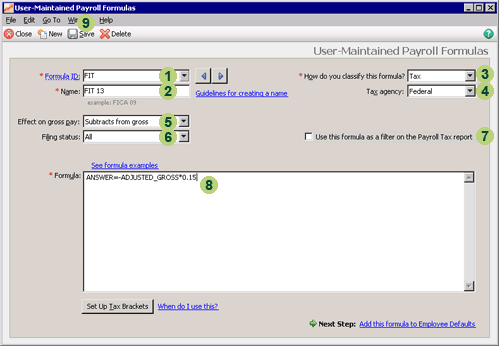Step Sage 50
field or icon
Action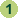Formula ID Carefully type the short identification code of the new formula you are creating. You should verify that the identification code does not already exist in Sage 50 otherwise you may accidentally alter another formula. Sage 50 helps you check this by displaying a drop down list of existing formulas and highlights the first formula starting with the identification code you enter as you type. Once you enter a new unique identification code no existing formula should be highlighted in this list.

Once you have checked your identification code is correct, move to the next step by clicking the OK icon at the bottom of the drop down list or pressing <Tab> or <Enter> on your keyboard.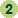Name Enter the name of the new formula you are creating.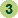How do you classify this formula? Select the type of the formula from the drop down list provided. It is important that you select the correct type so that the formula is used for its intended purpose in Sage 50.

If you are completing the Sage 50 Practice Set, you will only have to set up a tax formula. Note that the Tax agency field appears if you select Tax.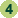Tax agency Select the type of government the tax formula applies to. You can either select Federal, State or Locality from the list of options available.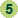Effect on gross pay Select the effect of the calculation on gross pay.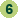Filing status Select the appropriate filing status.

If you are completing the Sage 50 Practice Set, you are asked to create a formula that is to be used for all employees so you should select All from the drop down list provided.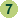Use this formula as a filter on the Payroll Tax report Click the box next to this item to enter a check mark if you want to use the formula as a filter on the payroll tax report.

If you are completing the Sage 50 Practice Set, just leave this box blank.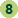Formula Enter one or more equations you use to calculate the result of the formula.

If you are completing the Sage 50 Practice Set, you will be given the payroll tax formula that you are required to enter into Sage 50. To find out how you can create a basic payroll equation, refer to the extra information below.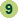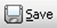Once you have entered the information in the User-Maintained Payroll Formulas window, remember to review your entries and click the Save icon to record the formula.

### Extra information on how to create a payroll formula in Sage 50

To create a formula in Sage 50, you are required to specify the variable that represents the result of the formula and the mathematical expresions that determine how that result is to be calculated. A payroll formula may contain one or more equations. A single-equation formula usually starts with the ANSWER variable that represents the result of the formula followed by an equal sign (=) and a mathematical expression that explains how that result is determined. The right side of the equation may show one or more of the commonly used Sage 50 identifiers, one or more mathematical operators (such as an asterisk * for the multiplication symbol), and one or more other constants and variables.

An important component of any mathematical expression will be the special identifiers that Sage 50 provides to represent important payroll variables. For example, Sage 50 provides an identifier known as ADJUSTED_GROSS which is the difference between the gross employee compensation and all allowable deductions. These identifiers can then be used to calculate other payroll variables such as benefits, deductions and taxation.

In the example below, an amount of tax is to be calculated using a Sage 50 identifier, a multiplication sign and a constant of 0.15 (being 15%). In addition, the right side of the equation starts with a minus sign (-) to show that the answer is a deduction from the payroll.